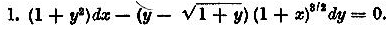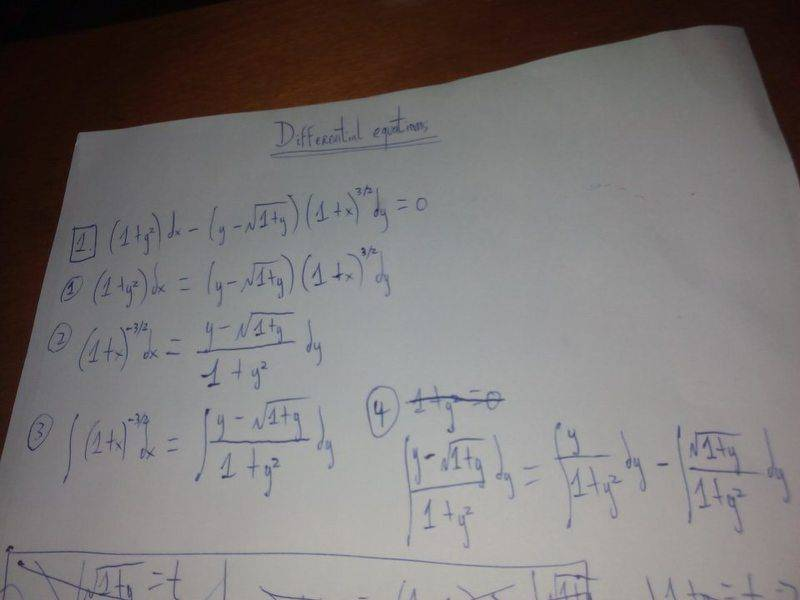# Simple differential equation

## Homework Statement

[/B]
Solve the differential equation## The Attempt at a Solution

[/B]I just can't integrate that (1+y)^(1/2)/(1+y^2)dy at the end... the other two integrals are trivial.

#### Attachments

•